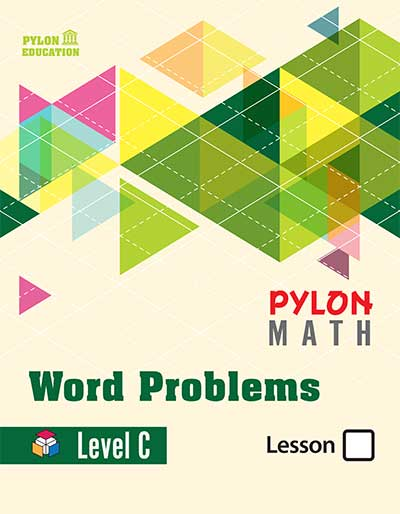# Word Problem Level C1. ### Place Values

• Place Values, Counting, and Order
• Ordinal Numbers
• Place values of 100, 1000
• Ten-thousands, Millions
• Comparing Numbers
• Rounding to Tens and Hundreds
• Number Sense

• Guess and Check
• Adding 2-digit, 3-digit, 4-digit Numbers
• Regrouping
• Minimum and Maximum of Range
• More and All, Easy Math
• Front-End Estimation
• Mental Math, Money, Critical Thinking
3. ### Subtraction

• Math Reasoning, Meaning of Subtraction
• Patterns, Estimating Differences
• Subtracting 2-digit, 3-digit Numbers
• Subtracting Across 0
• Subtracting 4-digit Numbers
• Analyzing Word Problems
• Easy Math, Subtracting Money
4. ### Money & Time

• Counting Coins, Money, Making Change
• Telling Time to the nearest 5 minutes
• Half Hour and Quarter Hour, A.M. and P.M.
• Elapsed Time, Units of Time
5. ### Multiplication

• Multiplication Concept
• Multiplication sentences
• Multiplication with 2 and 5
• Commutative Property
• Multiply with 0,1,2,3,5,9
• Analyze Word Problems
• Analyze Multiplication
• Work Backward
1. ### Multiplication & Patterns

• Word Problems, Multiples
• Making Plans
• Using Known Facts
• Multiples of 3,6,10,11,12
• Commutative Property
• Solving Strategies
2. ### Division

• Equal Groups
• Division by Subtractinng
• Equal Amounts
• Choosing Operations
• Dividing Big Numbers
• Dividing with 1 & 0
• Different Units, Month and Year
• Even and Odd Numbers
• Fact Family
• Balancing Scales, Unit Cost
3. ### Graphs

• Pictographs, Bar Graphs
• Line Graphs
• Making Predictions
• Analyzing Word Problems
• Exploring Graphs, Classifying Data
• Line Plots, Mean & Median
• Ordered Pairs, Patterns
4. ### Customary Units

• Length, Inches, Foot, Yard, Mile
• Capacity, Cup, Pint, Quart, Gallon
• Weight, Ounces and Pounds
• Changing Units, Metric Units
• Millimeter, Gram, Fahrenheit and Celsius
5. ### Geometric Figures

• Basic Figures, Angles, Lines
• Plane Figures, Triangles